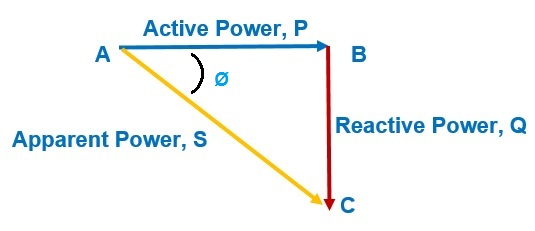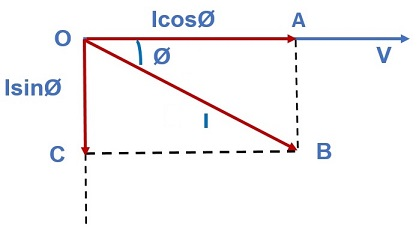# Power Triangle

Power Triangle is a right angled triangle whose sides represent the active, reactive and apparent power. Base, Perpendicular and Hypogenous of this right angled triangle denotes the Active, Reactive and Apparent power respectively.

This triangle is not a new concept but it is just the schematic representation of phasor diagram of inductive / capacitive load connected to a source. It is obtained by multiplying the circuit current I, active current (IcosØ) and reactive current (IsinØ) by voltage V. Multiplication of voltage V with circuit current I, active current IcosØ and reactive current IsinØ gives apparent power (S), active power (P) and reactive power (Q) respectively.The above figure shows a Power Triangle. Side AB, BC and AC represents P, Q and S respectively. The above triangle is obtained from the below phasor diagram:OA = Active Current

OC = Reactive Current

OB = Circuit Current

Power Triangle provides the relationship between the three quantities and following information

1) Active Power P, Reactive Power Q and Apparent Power S are related as

S = P + jQ

S2 = P2 + Q2

S = √(P2 + Q2)

2) Power Factor is defined as the cosine of angle between the voltage and current phasor. It is denoted as pf and given as

pf = cosØ

Thus, 0≤ pf ≤1

The value of power factor is 1 for pure resistance and 0 for pure inductor / capacitor.

3) The angle Ø between AB and AC in power triangle represents the angle by which current is lagging with voltage.

4) Active Power (P), Reactive Power (Q) and Apparent Power (S) are given as

P = VIcosØ

Q = VIsinØ

S = VI

5) The power factor can be obtained from the power triangle by taking the ratio of active power and apparent power, since cosØ = Base / Hypogenous = AB / AC

Power Factor = Active Power / Apparent Power

= P / S

6) Active, reactive and apparent power are measured in kW, kVAR and kVA respectively. kW, kVAR and kVA are related as

(kVA)2 = (kW)2 + (kVAR)2

### 2 thoughts on “Power Triangle”

1.mozzay says:

I concur with your sit, I have gain a lot. thanks

1.admin says:

Thank you! Please share if you like the post.

This site uses Akismet to reduce spam. Learn how your comment data is processed.